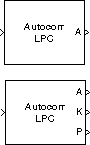# Autocorrelation LPC

Determine coefficients of Nth-order forward linear predictors

•Libraries:
DSP System Toolbox / Estimation / Linear Prediction

## Description

The Autocorrelation LPC block determines the coefficients of an N-step forward linear predictor for the time-series in each length-M input channel, u, by minimizing the prediction error in the least squares sense. A linear predictor is an FIR filter that predicts the next value in a sequence from the present and past inputs. This technique has applications in filter design, speech coding, spectral analysis, and system identification.

The Autocorrelation LPC block can output the prediction error for each channel as polynomial coefficients, reflection coefficients, or both. The block can also output the prediction error power for each channel.

## Ports

### Input

expand all

Specify the input u as an unoriented vector, column vector, or a matrix. Row vectors are not valid inputs. The block treats all M-by-N matrix inputs as N channels of length M.

Data Types: `single` | `double`

### Output

expand all

Polynomial coefficients generated when you set the Output(s) parameter to `A` or ```A and K```. For each input channel, port A outputs an (N+1)-by-1 column vector a = [1 a2a3 ... aN+1]T, containing the coefficients of an Nth-order moving average (MA) linear process that predicts the next value, ûM+1, in the input time-series.

`${\stackrel{^}{u}}_{M+1}=-\left({a}_{2}{u}_{M}\right)-\left({a}_{3}{u}_{M-1}\right)-...-\left({a}_{N+1}{u}_{M-N+1}\right)$`

#### Dependencies

To enable port A, set Output(s) to `A` or ```A and K```.

Data Types: `single` | `double`

Reflection coefficients generated when Output(s) is set to `K` or ```A and K```. For each input channel, port K outputs a length-N column vector whose elements are the prediction error reflection coefficients.

#### Dependencies

To enable port K, set Output(s) to `A` or ```A and K```.

Data Types: `single` | `double`

Prediction error power output at port P as a vector whose length is the number of input channels.

#### Dependencies

To enable port P, select the Output prediction error power (P) parameter.

Data Types: `single` | `double`

## Parameters

expand all

Specify the type of prediction coefficients output by the block. The block can output polynomial coefficients (`A`), reflection coefficients (`K`), or both (```A and K```).

When you set Output(s) to ```A and K```, the block enables port A and K and each port outputs its respective set of prediction coefficients for each channel.

Select this parameter to enable the output port `P`, which outputs the output prediction error power.

Select this parameter to inherit the prediction order N from the input dimensions.

Specify the prediction order N. Note that N must be a scalar with a value less than the length of the input channels or the block produces an error.

#### Dependencies

This parameter appears only when you do not select the Inherit prediction order from input dimensions parameter.

## Block Characteristics

 Data Types `double` | `single` Direct Feedthrough `no` Multidimensional Signals `no` Variable-Size Signals `no` Zero-Crossing Detection `no`

## Algorithms

The Autocorrelation LPC block computes the least squares solution to

`$\underset{i\in {\Re }^{n}}{\mathrm{min}}‖U\stackrel{˜}{a}-b‖$`

where $‖\cdot ‖$ indicates the 2-norm and

`$U=\left[\begin{array}{cccc}{u}_{1}& 0& \cdots & 0\\ {u}_{2}& {u}_{1}& \ddots & ⋮\\ ⋮& {u}_{2}& \ddots & 0\\ ⋮& ⋮& \ddots & {u}_{1}\\ ⋮& ⋮& ⋮& {u}_{2}\\ ⋮& ⋮& ⋮& ⋮\\ {u}_{M}& ⋮& ⋮& ⋮\\ 0& \ddots & ⋮& ⋮\\ ⋮& \ddots & \ddots & ⋮\\ 0& \cdots & 0& {u}_{M}\end{array}\right],\text{\hspace{0.17em}}\text{\hspace{0.17em}}\text{​}\text{​}\stackrel{˜}{a}=\left[\begin{array}{c}{a}_{2}\\ ⋮\\ {a}_{n+1}\end{array}\right],\text{\hspace{0.17em}}\text{\hspace{0.17em}}b=\left[\begin{array}{c}{u}_{2}\\ {u}_{3}\\ ⋮\\ {u}_{M}\\ 0\\ ⋮\\ 0\end{array}\right]$`

Solving the least squares problem via the normal equations

`${U}^{\ast }U\stackrel{˜}{a}={U}^{\ast }b$`

leads to the system of equations

`$\left[\begin{array}{cccc}{r}_{1}& {r}_{2}^{\ast }& \cdots & {r}_{n}^{\ast }\\ {r}_{2}& {r}_{1}& \ddots & ⋮\\ ⋮& \ddots & \ddots & {r}_{2}^{\ast }\\ {r}_{n}& \cdots & {r}_{2}& {r}_{1}\end{array}\right]\text{\hspace{0.17em}}\left[\begin{array}{c}{a}_{2}\\ {a}_{3}\\ ⋮\\ {a}_{n+1}\end{array}\right]\text{\hspace{0.17em}}=\left[\begin{array}{c}-{r}_{2}\\ -{r}_{3}\\ ⋮\\ -{r}_{n+1}\end{array}\right]$`

where r = [r1r2r3 ... rn+1]T is an autocorrelation estimate for u computed using the Autocorrelation block, and * indicates the complex conjugate transpose. The normal equations are solved in O(n2) operations by the Levinson-Durbin block.

Note that the solution to the LPC problem is very closely related to the Yule-Walker AR method of spectral estimation. In that context, the normal equations above are referred to as the Yule-Walker AR equations.

 Haykin, S. Adaptive Filter Theory. 3rd ed. Englewood Cliffs, NJ: Prentice Hall, 1996.

 Ljung, L. System Identification: Theory for the User. Englewood Cliffs, NJ: Prentice Hall, 1987. Pgs. 278-280.

 Proakis, J. and D. Manolakis. Digital Signal Processing. 3rd ed. Englewood Cliffs, NJ: Prentice-Hall, 1996.